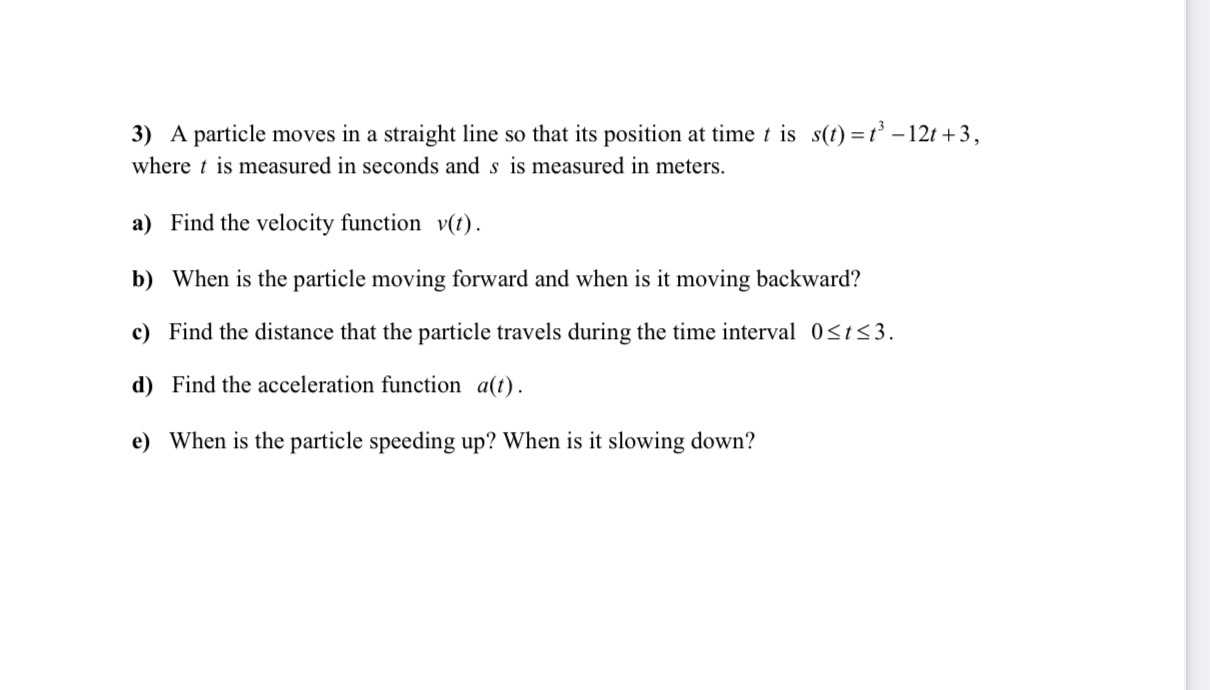### ¿Todavía tienes preguntas de matemáticas?

Pregunte a nuestros tutores expertos
Algebra
PreguntaA particle moves in a straight line so that its position at time $$t$$ is $$s ( t ) = t ^ { 3 } - 12 t + 3$$ ,  where $$t$$ is measured in seconds and $$s$$ is measured in meters.  a) Find the velocity function $$v ( t )$$ .  b) When is the particle moving forward and when is it moving backward?  d) Find the acceleration function $$a ( t )$$ .  e) When is the particle speeding up? When is it slowing down?

a) $$v(t)=3t^2-12$$

b) forward: $$v(t)>0\Rightarrow t>2$$

backward: $$v(t)<0\Rightarrow 0<t<2$$

c) $$d=|s(3)-s(0)|=9$$

d) $$a(t)=v'(t)=6t$$

e) $$t>0\Rightarrow a(t)>0\Rightarrow$$always speeding up

Solución
View full explanation on CameraMath App.